In six baskets, the seller has fruit. In individual baskets, there are only apples or just pears with the following number of fruits: 5,6,12,14,23 and 29. "If I sell this basket," the salesman thinks, "then I will have just as many apples as a pear." Which basket did the salesman think?

x =  29

### Step-by-step explanation:

```j5j6j12j14j23j29 :::H=0; J=89
**** K=5 ** H=0; J=84
**** K=6 ** H=0; J=83
**** K=12 ** H=0; J=77
**** K=14 ** H=0; J=75
**** K=23 ** H=0; J=66
**** K=29 ** H=0; J=60
h5j6j12j14j23j29 :::H=5; J=84
**** K=5 ** H=0; J=84
**** K=6 ** H=5; J=78
**** K=12 ** H=5; J=72
**** K=14 ** H=5; J=70
**** K=23 ** H=5; J=61
**** K=29 ** H=5; J=55
j5h6j12j14j23j29 :::H=6; J=83
**** K=5 ** H=6; J=78
**** K=6 ** H=0; J=83
**** K=12 ** H=6; J=71
**** K=14 ** H=6; J=69
**** K=23 ** H=6; J=60
**** K=29 ** H=6; J=54
h5h6j12j14j23j29 :::H=11; J=78
**** K=5 ** H=6; J=78
**** K=6 ** H=5; J=78
**** K=12 ** H=11; J=66
**** K=14 ** H=11; J=64
**** K=23 ** H=11; J=55
**** K=29 ** H=11; J=49
j5j6h12j14j23j29 :::H=12; J=77
**** K=5 ** H=12; J=72
**** K=6 ** H=12; J=71
**** K=12 ** H=0; J=77
**** K=14 ** H=12; J=63
**** K=23 ** H=12; J=54
**** K=29 ** H=12; J=48
h5j6h12j14j23j29 :::H=17; J=72
**** K=5 ** H=12; J=72
**** K=6 ** H=17; J=66
**** K=12 ** H=5; J=72
**** K=14 ** H=17; J=58
**** K=23 ** H=17; J=49
**** K=29 ** H=17; J=43
j5h6h12j14j23j29 :::H=18; J=71
**** K=5 ** H=18; J=66
**** K=6 ** H=12; J=71
**** K=12 ** H=6; J=71
**** K=14 ** H=18; J=57
**** K=23 ** H=18; J=48
**** K=29 ** H=18; J=42
h5h6h12j14j23j29 :::H=23; J=66
**** K=5 ** H=18; J=66
**** K=6 ** H=17; J=66
**** K=12 ** H=11; J=66
**** K=14 ** H=23; J=52
**** K=23 ** H=23; J=43
**** K=29 ** H=23; J=37
j5j6j12h14j23j29 :::H=14; J=75
**** K=5 ** H=14; J=70
**** K=6 ** H=14; J=69
**** K=12 ** H=14; J=63
**** K=14 ** H=0; J=75
**** K=23 ** H=14; J=52
**** K=29 ** H=14; J=46
h5j6j12h14j23j29 :::H=19; J=70
**** K=5 ** H=14; J=70
**** K=6 ** H=19; J=64
**** K=12 ** H=19; J=58
**** K=14 ** H=5; J=70
**** K=23 ** H=19; J=47
**** K=29 ** H=19; J=41
j5h6j12h14j23j29 :::H=20; J=69
**** K=5 ** H=20; J=64
**** K=6 ** H=14; J=69
**** K=12 ** H=20; J=57
**** K=14 ** H=6; J=69
**** K=23 ** H=20; J=46
**** K=29 ** H=20; J=40 OK OK OK
h5h6j12h14j23j29 :::H=25; J=64
**** K=5 ** H=20; J=64
**** K=6 ** H=19; J=64
**** K=12 ** H=25; J=52
**** K=14 ** H=11; J=64
**** K=23 ** H=25; J=41
**** K=29 ** H=25; J=35
j5j6h12h14j23j29 :::H=26; J=63
**** K=5 ** H=26; J=58
**** K=6 ** H=26; J=57
**** K=12 ** H=14; J=63
**** K=14 ** H=12; J=63
**** K=23 ** H=26; J=40
**** K=29 ** H=26; J=34
h5j6h12h14j23j29 :::H=31; J=58
**** K=5 ** H=26; J=58
**** K=6 ** H=31; J=52
**** K=12 ** H=19; J=58
**** K=14 ** H=17; J=58
**** K=23 ** H=31; J=35
**** K=29 ** H=31; J=29
j5h6h12h14j23j29 :::H=32; J=57
**** K=5 ** H=32; J=52
**** K=6 ** H=26; J=57
**** K=12 ** H=20; J=57
**** K=14 ** H=18; J=57
**** K=23 ** H=32; J=34
**** K=29 ** H=32; J=28
h5h6h12h14j23j29 :::H=37; J=52
**** K=5 ** H=32; J=52
**** K=6 ** H=31; J=52
**** K=12 ** H=25; J=52
**** K=14 ** H=23; J=52
**** K=23 ** H=37; J=29
**** K=29 ** H=37; J=23
j5j6j12j14h23j29 :::H=23; J=66
**** K=5 ** H=23; J=61
**** K=6 ** H=23; J=60
**** K=12 ** H=23; J=54
**** K=14 ** H=23; J=52
**** K=23 ** H=0; J=66
**** K=29 ** H=23; J=37
h5j6j12j14h23j29 :::H=28; J=61
**** K=5 ** H=23; J=61
**** K=6 ** H=28; J=55
**** K=12 ** H=28; J=49
**** K=14 ** H=28; J=47
**** K=23 ** H=5; J=61
**** K=29 ** H=28; J=32
j5h6j12j14h23j29 :::H=29; J=60
**** K=5 ** H=29; J=55
**** K=6 ** H=23; J=60
**** K=12 ** H=29; J=48
**** K=14 ** H=29; J=46
**** K=23 ** H=6; J=60
**** K=29 ** H=29; J=31
h5h6j12j14h23j29 :::H=34; J=55
**** K=5 ** H=29; J=55
**** K=6 ** H=28; J=55
**** K=12 ** H=34; J=43
**** K=14 ** H=34; J=41
**** K=23 ** H=11; J=55
**** K=29 ** H=34; J=26
j5j6h12j14h23j29 :::H=35; J=54
**** K=5 ** H=35; J=49
**** K=6 ** H=35; J=48
**** K=12 ** H=23; J=54
**** K=14 ** H=35; J=40
**** K=23 ** H=12; J=54
**** K=29 ** H=35; J=25
h5j6h12j14h23j29 :::H=40; J=49
**** K=5 ** H=35; J=49
**** K=6 ** H=40; J=43
**** K=12 ** H=28; J=49
**** K=14 ** H=40; J=35
**** K=23 ** H=17; J=49
**** K=29 ** H=40; J=20
j5h6h12j14h23j29 :::H=41; J=48
**** K=5 ** H=41; J=43
**** K=6 ** H=35; J=48
**** K=12 ** H=29; J=48
**** K=14 ** H=41; J=34
**** K=23 ** H=18; J=48
**** K=29 ** H=41; J=19
h5h6h12j14h23j29 :::H=46; J=43
**** K=5 ** H=41; J=43
**** K=6 ** H=40; J=43
**** K=12 ** H=34; J=43
**** K=14 ** H=46; J=29
**** K=23 ** H=23; J=43
**** K=29 ** H=46; J=14
j5j6j12h14h23j29 :::H=37; J=52
**** K=5 ** H=37; J=47
**** K=6 ** H=37; J=46
**** K=12 ** H=37; J=40
**** K=14 ** H=23; J=52
**** K=23 ** H=14; J=52
**** K=29 ** H=37; J=23
h5j6j12h14h23j29 :::H=42; J=47
**** K=5 ** H=37; J=47
**** K=6 ** H=42; J=41
**** K=12 ** H=42; J=35
**** K=14 ** H=28; J=47
**** K=23 ** H=19; J=47
**** K=29 ** H=42; J=18
j5h6j12h14h23j29 :::H=43; J=46
**** K=5 ** H=43; J=41
**** K=6 ** H=37; J=46
**** K=12 ** H=43; J=34
**** K=14 ** H=29; J=46
**** K=23 ** H=20; J=46
**** K=29 ** H=43; J=17
h5h6j12h14h23j29 :::H=48; J=41
**** K=5 ** H=43; J=41
**** K=6 ** H=42; J=41
**** K=12 ** H=48; J=29
**** K=14 ** H=34; J=41
**** K=23 ** H=25; J=41
**** K=29 ** H=48; J=12
j5j6h12h14h23j29 :::H=49; J=40
**** K=5 ** H=49; J=35
**** K=6 ** H=49; J=34
**** K=12 ** H=37; J=40
**** K=14 ** H=35; J=40
**** K=23 ** H=26; J=40
**** K=29 ** H=49; J=11
h5j6h12h14h23j29 :::H=54; J=35
**** K=5 ** H=49; J=35
**** K=6 ** H=54; J=29
**** K=12 ** H=42; J=35
**** K=14 ** H=40; J=35
**** K=23 ** H=31; J=35
**** K=29 ** H=54; J=6
j5h6h12h14h23j29 :::H=55; J=34
**** K=5 ** H=55; J=29
**** K=6 ** H=49; J=34
**** K=12 ** H=43; J=34
**** K=14 ** H=41; J=34
**** K=23 ** H=32; J=34
**** K=29 ** H=55; J=5
h5h6h12h14h23j29 :::H=60; J=29
**** K=5 ** H=55; J=29
**** K=6 ** H=54; J=29
**** K=12 ** H=48; J=29
**** K=14 ** H=46; J=29
**** K=23 ** H=37; J=29
**** K=29 ** H=60; J=0
j5j6j12j14j23h29 :::H=29; J=60
**** K=5 ** H=29; J=55
**** K=6 ** H=29; J=54
**** K=12 ** H=29; J=48
**** K=14 ** H=29; J=46
**** K=23 ** H=29; J=37
**** K=29 ** H=0; J=60
h5j6j12j14j23h29 :::H=34; J=55
**** K=5 ** H=29; J=55
**** K=6 ** H=34; J=49
**** K=12 ** H=34; J=43
**** K=14 ** H=34; J=41
**** K=23 ** H=34; J=32
**** K=29 ** H=5; J=55
j5h6j12j14j23h29 :::H=35; J=54
**** K=5 ** H=35; J=49
**** K=6 ** H=29; J=54
**** K=12 ** H=35; J=42
**** K=14 ** H=35; J=40
**** K=23 ** H=35; J=31
**** K=29 ** H=6; J=54
h5h6j12j14j23h29 :::H=40; J=49
**** K=5 ** H=35; J=49
**** K=6 ** H=34; J=49
**** K=12 ** H=40; J=37
**** K=14 ** H=40; J=35
**** K=23 ** H=40; J=26
**** K=29 ** H=11; J=49
j5j6h12j14j23h29 :::H=41; J=48
**** K=5 ** H=41; J=43
**** K=6 ** H=41; J=42
**** K=12 ** H=29; J=48
**** K=14 ** H=41; J=34
**** K=23 ** H=41; J=25
**** K=29 ** H=12; J=48
h5j6h12j14j23h29 :::H=46; J=43
**** K=5 ** H=41; J=43
**** K=6 ** H=46; J=37
**** K=12 ** H=34; J=43
**** K=14 ** H=46; J=29
**** K=23 ** H=46; J=20
**** K=29 ** H=17; J=43
j5h6h12j14j23h29 :::H=47; J=42
**** K=5 ** H=47; J=37
**** K=6 ** H=41; J=42
**** K=12 ** H=35; J=42
**** K=14 ** H=47; J=28
**** K=23 ** H=47; J=19
**** K=29 ** H=18; J=42
h5h6h12j14j23h29 :::H=52; J=37
**** K=5 ** H=47; J=37
**** K=6 ** H=46; J=37
**** K=12 ** H=40; J=37
**** K=14 ** H=52; J=23
**** K=23 ** H=52; J=14
**** K=29 ** H=23; J=37
j5j6j12h14j23h29 :::H=43; J=46
**** K=5 ** H=43; J=41
**** K=6 ** H=43; J=40
**** K=12 ** H=43; J=34
**** K=14 ** H=29; J=46
**** K=23 ** H=43; J=23
**** K=29 ** H=14; J=46
h5j6j12h14j23h29 :::H=48; J=41
**** K=5 ** H=43; J=41
**** K=6 ** H=48; J=35
**** K=12 ** H=48; J=29
**** K=14 ** H=34; J=41
**** K=23 ** H=48; J=18
**** K=29 ** H=19; J=41
j5h6j12h14j23h29 :::H=49; J=40
**** K=5 ** H=49; J=35
**** K=6 ** H=43; J=40
**** K=12 ** H=49; J=28
**** K=14 ** H=35; J=40
**** K=23 ** H=49; J=17
**** K=29 ** H=20; J=40 OK OK OK
h5h6j12h14j23h29 :::H=54; J=35
**** K=5 ** H=49; J=35
**** K=6 ** H=48; J=35
**** K=12 ** H=54; J=23
**** K=14 ** H=40; J=35
**** K=23 ** H=54; J=12
**** K=29 ** H=25; J=35
j5j6h12h14j23h29 :::H=55; J=34
**** K=5 ** H=55; J=29
**** K=6 ** H=55; J=28
**** K=12 ** H=43; J=34
**** K=14 ** H=41; J=34
**** K=23 ** H=55; J=11
**** K=29 ** H=26; J=34
h5j6h12h14j23h29 :::H=60; J=29
**** K=5 ** H=55; J=29
**** K=6 ** H=60; J=23
**** K=12 ** H=48; J=29
**** K=14 ** H=46; J=29
**** K=23 ** H=60; J=6
**** K=29 ** H=31; J=29
j5h6h12h14j23h29 :::H=61; J=28
**** K=5 ** H=61; J=23
**** K=6 ** H=55; J=28
**** K=12 ** H=49; J=28
**** K=14 ** H=47; J=28
**** K=23 ** H=61; J=5
**** K=29 ** H=32; J=28
h5h6h12h14j23h29 :::H=66; J=23
**** K=5 ** H=61; J=23
**** K=6 ** H=60; J=23
**** K=12 ** H=54; J=23
**** K=14 ** H=52; J=23
**** K=23 ** H=66; J=0
**** K=29 ** H=37; J=23
j5j6j12j14h23h29 :::H=52; J=37
**** K=5 ** H=52; J=32
**** K=6 ** H=52; J=31
**** K=12 ** H=52; J=25
**** K=14 ** H=52; J=23
**** K=23 ** H=29; J=37
**** K=29 ** H=23; J=37
h5j6j12j14h23h29 :::H=57; J=32
**** K=5 ** H=52; J=32
**** K=6 ** H=57; J=26
**** K=12 ** H=57; J=20
**** K=14 ** H=57; J=18
**** K=23 ** H=34; J=32
**** K=29 ** H=28; J=32
j5h6j12j14h23h29 :::H=58; J=31
**** K=5 ** H=58; J=26
**** K=6 ** H=52; J=31
**** K=12 ** H=58; J=19
**** K=14 ** H=58; J=17
**** K=23 ** H=35; J=31
**** K=29 ** H=29; J=31
h5h6j12j14h23h29 :::H=63; J=26
**** K=5 ** H=58; J=26
**** K=6 ** H=57; J=26
**** K=12 ** H=63; J=14
**** K=14 ** H=63; J=12
**** K=23 ** H=40; J=26
**** K=29 ** H=34; J=26
j5j6h12j14h23h29 :::H=64; J=25
**** K=5 ** H=64; J=20
**** K=6 ** H=64; J=19
**** K=12 ** H=52; J=25
**** K=14 ** H=64; J=11
**** K=23 ** H=41; J=25
**** K=29 ** H=35; J=25
h5j6h12j14h23h29 :::H=69; J=20
**** K=5 ** H=64; J=20
**** K=6 ** H=69; J=14
**** K=12 ** H=57; J=20
**** K=14 ** H=69; J=6
**** K=23 ** H=46; J=20
**** K=29 ** H=40; J=20
j5h6h12j14h23h29 :::H=70; J=19
**** K=5 ** H=70; J=14
**** K=6 ** H=64; J=19
**** K=12 ** H=58; J=19
**** K=14 ** H=70; J=5
**** K=23 ** H=47; J=19
**** K=29 ** H=41; J=19
h5h6h12j14h23h29 :::H=75; J=14
**** K=5 ** H=70; J=14
**** K=6 ** H=69; J=14
**** K=12 ** H=63; J=14
**** K=14 ** H=75; J=0
**** K=23 ** H=52; J=14
**** K=29 ** H=46; J=14
j5j6j12h14h23h29 :::H=66; J=23
**** K=5 ** H=66; J=18
**** K=6 ** H=66; J=17
**** K=12 ** H=66; J=11
**** K=14 ** H=52; J=23
**** K=23 ** H=43; J=23
**** K=29 ** H=37; J=23
h5j6j12h14h23h29 :::H=71; J=18
**** K=5 ** H=66; J=18
**** K=6 ** H=71; J=12
**** K=12 ** H=71; J=6
**** K=14 ** H=57; J=18
**** K=23 ** H=48; J=18
**** K=29 ** H=42; J=18
j5h6j12h14h23h29 :::H=72; J=17
**** K=5 ** H=72; J=12
**** K=6 ** H=66; J=17
**** K=12 ** H=72; J=5
**** K=14 ** H=58; J=17
**** K=23 ** H=49; J=17
**** K=29 ** H=43; J=17
h5h6j12h14h23h29 :::H=77; J=12
**** K=5 ** H=72; J=12
**** K=6 ** H=71; J=12
**** K=12 ** H=77; J=0
**** K=14 ** H=63; J=12
**** K=23 ** H=54; J=12
**** K=29 ** H=48; J=12
j5j6h12h14h23h29 :::H=78; J=11
**** K=5 ** H=78; J=6
**** K=6 ** H=78; J=5
**** K=12 ** H=66; J=11
**** K=14 ** H=64; J=11
**** K=23 ** H=55; J=11
**** K=29 ** H=49; J=11
h5j6h12h14h23h29 :::H=83; J=6
**** K=5 ** H=78; J=6
**** K=6 ** H=83; J=0
**** K=12 ** H=71; J=6
**** K=14 ** H=69; J=6
**** K=23 ** H=60; J=6
**** K=29 ** H=54; J=6
j5h6h12h14h23h29 :::H=84; J=5
**** K=5 ** H=84; J=0
**** K=6 ** H=78; J=5
**** K=12 ** H=72; J=5
**** K=14 ** H=70; J=5
**** K=23 ** H=61; J=5
**** K=29 ** H=55; J=5
h5h6h12h14h23h29 :::H=89; J=0
**** K=5 ** H=84; J=0
**** K=6 ** H=83; J=0
**** K=12 ** H=77; J=0
**** K=14 ** H=75; J=0
**** K=23 ** H=66; J=0
**** K=29 ** H=60; J=0
```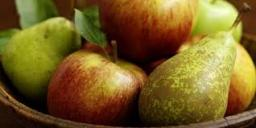Did you find an error or inaccuracy? Feel free to write us. Thank you!## Related math problems and questions: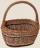Determine how many apples are in baskets when in the first basket are 4 apples, and in any other is 29 apples more than the previous, and we have eight baskets.
• Apples and pearsMom divided 24 apples and 15 pears to children. Each child received the same number of apples and pears - same number as his siblings. How many apples (j=?) and pears (h=?) received each child?
• Apples and pearsApples cost 50 cents piece, pears 60 cents piece, bananas cheaper than pears. Grandma bought 5 pieces of fruit. There was only one banana, and I paid 2 euros 75 cents. How many apples and how many pears?
• FruitsIn the shop sell 4 kinds of fruits. How many ways can we buy three pieces of fruit?Grandfather gathered apples into baskets. He filled one large and three small baskets. Small baskets are identical and each can hold 3 kg of apples less than in a large basket. In small baskets together 3 kg of apples over a large basket. How many kilogra
• A man 3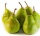A man buys a box of fruits containing 286 fruits out of these 1/2 are apples and the rest are pears. 4/13 of the pears are rotten. He sells the good pears at rupees 4 1/11 each. How much money does he receive on selling the good pears?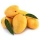A basket contains three types of fruits weighing 87/4 kg in all. If 23/4 kilograms of these are oranges, 48/7 kg are mangoes, and the rest are apples. What is the weight of the apples in the basket?A basket is full of 50 different kinds of fruits that has a ratio of 2:3:5. If the fruits are apple, mango, and guava, how many guavas are there?
• A farmer 3A farmer sells 9.3 kg of pears and apples at the farmers market.3/4 of this weight is pears, and the rest is apples. How many kilograms of apples did she sell at the farmers market.The fruit basket is five times heavier than the empty basket. Filled is 20kg heavier than empty. Determine the weight of the fruit in the basket.
• Fruit equation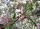Find how many peaches could be bought instead of buying one melon, one pear, one apple and two plums if we know that: • Melon and plum cost the same as three apples • if we subtract the price of a pear from the price of peach, we get the price of plum • T
• In the orchardIn the orchard, they planted 25 apple trees, 20 pears, 15 plums and 40 marbles. A strong late frost, however, destroyed a fifth of all new trees. Unfortunately, it was all the trees of one kind of fruit. What is the probability that the plums have died ou
• Apples 14Maya bought 18 apples. She used 50% of the apples to make apple pies, and 1/3 of the apples were mixed with other fruits to make the salad. How many apples were left?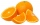If there are 7 apples and 5 oranges in the basket then what fraction of oranges are there in the fruit basket?How many different ways can three people divide 7 pears and 5 apples?The weight of the empty fruit basket is ¾ kg. We added ½ kg apples and 5/4 kg guavas. What will be the total weight of the fruit basket?There were pears in the basket, I took two-fifths of them, and left six in the basket. How many pears did I take?